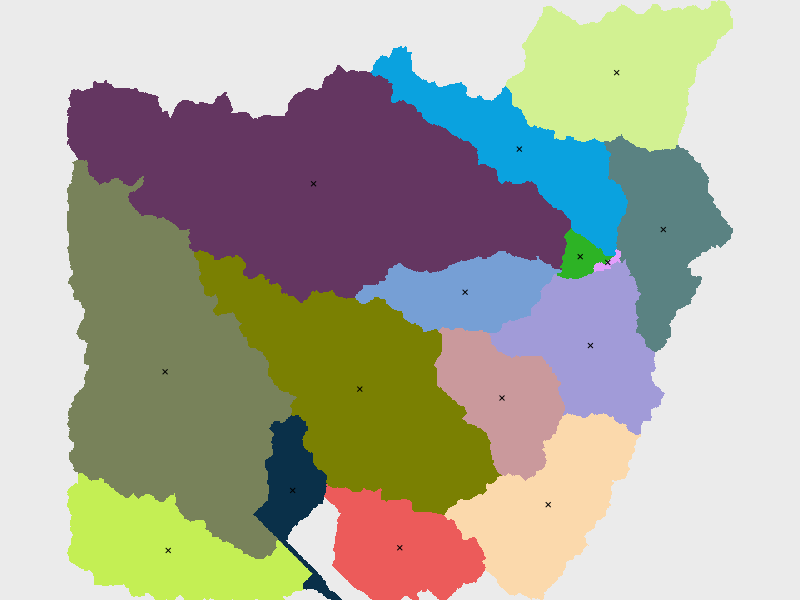## NAME

r.centroids - Creates vector map of centroids from raster of "clumps".

## KEYWORDS

raster, centroid, clumps, vector, centerpoint

## SYNOPSIS

r.centroids
r.centroids --help
r.centroids input=name output=name [--overwrite] [--help] [--verbose] [--quiet] [--ui]

### Flags:

--overwrite
Allow output files to overwrite existing files
--help
Print usage summary
--verbose
Verbose module output
--quiet
Quiet module output
--ui
Force launching GUI dialog

### Parameters:

input=name [required]
Raster map of clumps, clusters of same-valued pixels
output=name [required]
Name for output vector map

## DESCRIPTION

r.centroids is a wrapper function for r.volume that computes the center point of raster clumps of data (neighboring, same-valued pixels). The centroids will always fall within the clump so they may not be the true, mathematical centroid. The centroids will always fall at the center of a pixel.

r.centroids requires an input raster containing clumps of data such as the output of r.clump.

## EXAMPLES

Find the centroids of the basins map (North Carolina sample dataset). First, set computational area.
```g.region raster=basin_50K
```
Then, compute the centroids.
```r.centroids input=basin_50K output=centroids50K
```